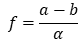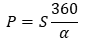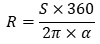# Calculation of Earth‘s model using results of Struve Geodetic Arc

How meridian measurements alow to determine the size of the Earth

Since the time of Eratosthenes, attempts to estimate the size and shape of the Earth have led to the realisation that the shape of the Earth essentially follows a mathematical model - an ellipsoid or spheroid, made up of a major semi-axis a, and a minor semi-axis b (Figure 1).Figure 1. Elipsoide of the Earth

Eratosthenes calculated that if we could measure the Earth's ellipsoid as an irregular circle of 360 degrees, and if we were able to measure the length of one degree in the circle, we would have the entire circumference of the Earth. However, the problem is not so simple because, as can be seen from Figure 1, the radius of curvature of the Earth's ellipsoid is variable, i.e. the Earth varies unequally at the equator and on the North or South axes. It is therefore necessary to determine the angle of curvature of the ellipsoid, α, as close as possible to the actual surface of the Earth by ground and astronomical measurements, and once this has been determined, we can obtain the flattening of the Earth, which is the difference between the two axes divided by the angle of curvatureAlso the term eccentricity , a positive real number expressing the characteristics of a tendency:

In mathematics, the eccentricity term, non negative real number of conic section, applies uniquely characterizing the Earth shape. It is defined as follwing:The meridian radius of curvature can be shown to be equal toThe arc length of an section S of the meridian from the equator to latitude φ is dm = M(φ) dφ is cakculated as following.Expression for a given meridian segment:It should be considered that the shape of the Earth causes critical differences in flattening on the Earth's axes and at the equator. If we try to measure the distance along the meridian at the same angle of change on the axes of the Earth's ellipsoid and at the equator (Figure 2) , the distance S1 would be greater than the distance S2.Figure 2. Difference in flatenning of the Earth.

It is clear from the above example that if we could determine the distance on the Earth's meridian and find out how many degrees of latitude this distance covers on the Earth's ellipsoid, we would subtract 1 degree on the meridian, and multiply it by 360 to get the entire circumference of the Earth. The longer meridian length S is, the closer to the real flattening of the Earth we could apply to the curvature (Figure 3). Once the meridian length S is determined to 1 degree, the circumference of the Earth is easy to calculate as following:,

Also the radius of the Earth -.Figure 3. Length of meridian on elipsoide

Determining the meridian by a triangulation arc chain

The triangulation method, introduced in the 17th century, made it possible to measure long distances more accurately by eliminating elevation issues on the ground. The Struve geodetic arc measurements were based on a modified triangulation method, introduced by the Dutch scientist Willebrord Snel van Royen. Triangular segments were projected in the geodetic chain so, that the sides (baseline) of one triangle in both sides of the segment was surveyed in high precision on the ground, as shown by a red double line (Figure 4).Fig. 4 Triangulation chain along a meridian.

The triangulation measurements were made by surveying on the ground all the angles of the triangles of the chain, and the distances of each side were calculated applying the trigonometric formula of sinus theorem (Figure 5). To ensure control of the calculations, precise measurements of the distance of one triangle side was again surveyed in the field at the end of each segment (Figure 4).Fig 5. Applies of principles of sinus trigonometric theory.

In total, 10 sides (baselines) have been accurately surveyed in the Struve geodetic arc, and astronomical observations have been made at 13 points to get the positioning of the points in space and to calculate the earth's radius of curvature by calculating astronomical latitudes and the astronomical azimuths, i.e. the directions to the neighbouring points. The astronomical observations were carried out using high-precision telescopes. The results have been achieved based on positioning of stars at certain timing. The astronomical parameters were adjusted using astronomical reference books.

Triangulation measurements were not necessarily taken exactly on the meridian, as the terrain and vegetation often made this impossible. Once the results of the calculations had been processed and the astronomical latitudes of each point on the chain had been calculated, they were mathematically projected onto the meridian line, thus fixing the longitude of the meridian. The Struve geodetic arc is 2822 km long, with Fuglenes (Norway) as the northernmost point and Staro Nekrasovka as the southernmost. The points of the Arc in prof. F.G.W. Struve's report were projected onto the so-called Tartu meridian, 26 degrees and 43 minutes East of Greenwich.

The first practical application of the data of Struve geodetic arc were utilized by Friedrich Wilhelm Bessel, who calculated the parameters of the so-called Bessel ellipsoid: whom the radius from the center of the Earth to the equator a = 6 337 397 m, Earth's flattening, α = 1 / 299,15. The Bessel ellipsoid has served for more than 100 years as a reliable basis for the Earth model in a number of applied geodetic coordinate systems.Fig. 6 Section of meridian length on an ellipsoid.

When considering the spherical transformation of the latitudes and longitudes of the Struve geodetic arc, several aspects of the mathematical analysis need to be highlighted. Consider two points on a spherical surface, A with latitude φ1 and longitude λ1, and B with latitude φ2 and longitude λ2 (Figure 6). The connecting spherical segment (from A to B) is AB with the length S12 and azimuths α1 and α2 at both ends. Once we have determined A, α1, and S12, we can solve the geodesic problem by determining the parameters of the next point B and its azimuth in the north direction α2.

Using the given example, by solving a spherical trigonometrical issue where the meridian's north direction is known, we can calculate the triangle NAB by applying the mathematical trigonometric (triangle) formula.,

Where a sum of angles of the triangle always isFig. 7. Element of a meridian projection

For the change in curvature ρ on the surface of an ellipsoid, F.W. Bessel derives a relationship between the change in azimuth α, the change in longitude ds and the change in latitude dφ (Figure 7).An expression of meridian section is:Arranged by dr. Saulius Urbanas.

---

References:

Bessel, F. W. (2010) . Translated by Karney, C. F. F.; Deakin, R. E. "The calculation of longitude and latitude from geodesic measurements". Astronomische Nachrichten. 331 (8): 852–861. arXiv:0908.1824

Karney, C. F. F. (2013). "Algorithms for geodesics". Journal of Geodesy. 87 (1): 43–55. arXiv:1109.4448. Bibcode:2013 Journal of Geodesy, Volume 87.

Smith J. R: The Struve Geodetic Arc. International Institution for History of Surveying & Measurement. FIG, 2005.

Struve W. 1957. Arc of Meridian. Moscow

Struve, W.: The arc of the meridian of 25∘20′ between the Danube and the Arctic Sea, measured from 1816 to 1855 under the direction of K. Tenner, N. H. Selander, Ch. Hansteen, and W. Struve.

Struve, W.: Géodésie. Sur la jonction de deux mesures de degres executees en Russie, Bibliotheque Universelle, des Sciences, Belles-Lettres et Arts. Sciences et arts. Faisant Suite a la Biblioteque Britannique, Redigee a Geneve